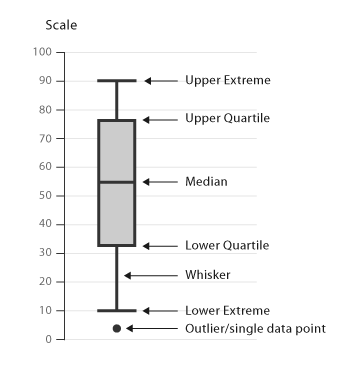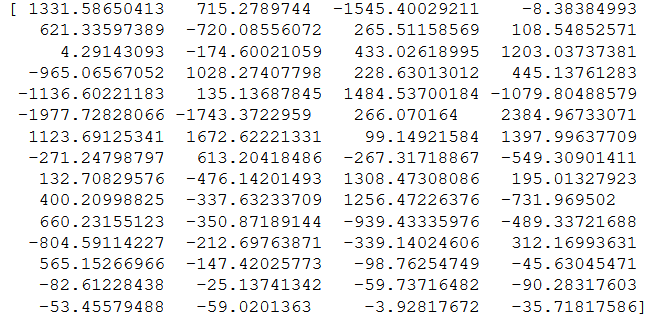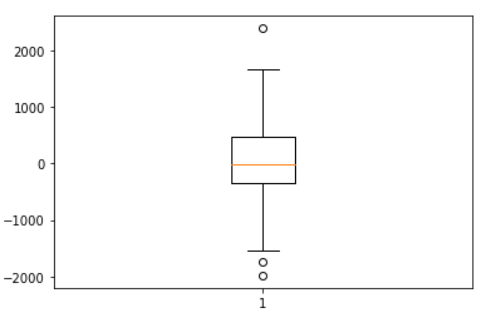# Box and Whisker Plot

## Introduction

Importance of Data Visualization

Data Visualization is the process of taking raw data and transforming it into graphs, charts, or images to derive meaningful insights from it.

It enables us to gain a qualitative understanding of the data by helping us identify new patterns, trends, outliers, and much more from the data. We can demonstrate the key relationships in the data and the numerical measures in different plots and graphs, which can help us and the stakeholders gain an overall sense of the data.

Thousands of rows of data can be easily visualized in graphs and pie charts.  It would be straightforward for a product-based company to understand how their product is performing comparatively in different regions by visualizing its sales in a pie chart(in percentage sold in each region) rather than looking at only the sheer numbers of the sales for each region.

Therefore, Data Visualization is a crucial technique for businesses. Data can be visualized in a variety of ways with the help of plots such as the line plot, scatter plot, box and whisker plot, histogram plot, pie charts, and much more.

In this blog, we will study the box and whisker plot.

## Terminologies

Before we dive deep into the boxplot, Let us learn about some statistical terminologies which are essential for the complete understanding of the plot.

Median
Median is the value separating the higher half from the lower half of the data sample, i.e., it is the middle value of the data sample.

Quartiles
Like the median divides the data so that 50% of the data lies below the median and the other 50% lies above the median, quartiles divide the data into quarters. We will learn about some essential terminologies related to quartiles depicted in the box and whisker plot.

1. First Quartile(Q1 or 25th percentile) - The lowest 25% of the numbers lie in the first quarter.  The first quartile(Q1) value is the median of the lower half of the dataset.
2. Second Quartile(Q2 or 50th percentile)-  The 25.1% to 50%(up to the median) of the number lie in the second quarter. The second quartile (Q2) value is the median itself.
3. Third Quartile(Q3 or 75th percentile)- The  50.1% to 75% of the numbers lie in the third quarter. The Third Quartile(Q3) value is the median of the upper half of the dataset. The highest 25% of the numbers lie in the fourth quarter.
4. Interquartile Range(IQR)- It is the difference between the third quartile (Q3) and the first quartile (Q1).   - IQR= Q3-Q1
5. Outliers- Outliers are all the data points that do not lie between the range (Q1-1.5*IQR) and (Q3+1.5*IQR).
6. Minimum(Q0 or 0th percentile)- It is the lowest data point (excluding any outliers).
7. Maximum(Q4 or 100th percentile)- It is the highest data point (excluding any outliers)

## Box and Whisker Plot

A box plot is a graph that gives you a good indication of how the values in the data are distributed as it displays data distribution through their quartiles.Source: datavizcatalogue.com

This image is a representation of a box plot on a data sample.

The y-axis shows the data range and the labels of the values you are graphing. A boxplot can be represented horizontally or vertically( the x-axis would show the data range in a horizontal plot).

The lines extending from the box are called the "whiskers." The whisker below the Lower quartile (Q1) ends at the minimum value in the dataset (Q0 or 0th percentile), and the whisker above the upper quartile (Q3) ends at the maximum value in the dataset (Q4 or 100th percentile). A single point on the plot represents the outliers.

The box is drawn from the first quartile (Q1) to the third quartile (Q3). The line between the box represents the Median of the data, and the length of the box is the Interquartile Range(IQR).

We will see an example on how to create a box plot for a random data sample in python. We will also learn about Matplotlib, a plotting library in python.

## Box Plots in Python

Introduction to Matplotlib

Matplotlib is a visualization library in python that offers us a wide variety of visualizations such as line, bar, scatter, histogram, boxplot, and many more. We can create beautiful visual charts and graphs with ease and define our own custom labels for the axes, the plot's title, the color of the plot, and a lot more.

We can easily customize our draw and customize our plots using the functions under the pyplot module in matplotlib.

``import matplotlib.pyplot as plt``

The above python code shows how we can import the pyplot and give it an alias called plt to use the functions available under the module.

Now we will learn how to create box plots using matplotlib.

Boxplots in Python

Let us start by importing libraries matplotlib and numpy, which we will be using to create the random data.

``````import matplotlib.pyplot as plt
import numpy as np``````

Now let's create some random data.

``````np.random.seed(10) #Same random data on each run
intitial_data=np.random.randn(50)*100 #The initial random data
lower_values = np.random.rand(10) * -1000 # Some low values which can act as outliers
data = np.concatenate((initial_data,lower_values))``````

This is our final data in the numpy array. (The values generated are random and would be different for you).Now let us utilize the boxplot function in pyplot to construct the box plot for the above data.

``````plt.boxplot(data) # Creating plot
plt.show() #Show plot``````

The image below is the final output plot.If we want to save this plot, we can save it by using the command:-

``plt.savefig("boxplot.png")``

This will save the image in the current working directory with the name "boxplot."

1. Why do we use box plots?
Box plots are helpful as they provide a visual summary of the data sample, enabling us to identify mean value and the following:-
Skewness-  It can show if the data sample is normally distributed or skewed. If the median is at the middle, the data is symmetric; else, if it is more towards the left or right, the data sample is skewed.
Dispersion- It helps us to identify the extent to which the data is spread. The maximum and minimum value of the whiskers gives us an idea about how the data is spread and its range.
Outliers-  We can quickly identify the outliers in our data sample by looking at the box plot.
Also, in comparison to histograms, they have the advantage of taking up less space, which is useful when comparing distributions between many groups or datasets.

2. How can we customize the boxplot in python?
Matplotlib's pyplot offers a lot of customization options for box plots. We can customize the plot to be horizontal or vertical, decide the position and width of the boxes, change the styling, apply labels to the axes, and much more. I would recommend that you look at the documentation of the boxplot to learn about all the possible customization.
matplotlib.pyplot.boxplot — Matplotlib 3.1.2 documentation

3. Is Matplotlib the only library to create box plots in python?
No, there are other ways to create box plots in python. We can create box plots in pandas or by utilizing another visualization library in python called seaborn.

## Key takeaways

In this blog, we learned about data visualization, understood box plots, and implemented them in python. I hope this article gave you enough knowledge to continue to learn more and more about visualizations and gave you a sense of where box plots can act as a useful visualization tool.